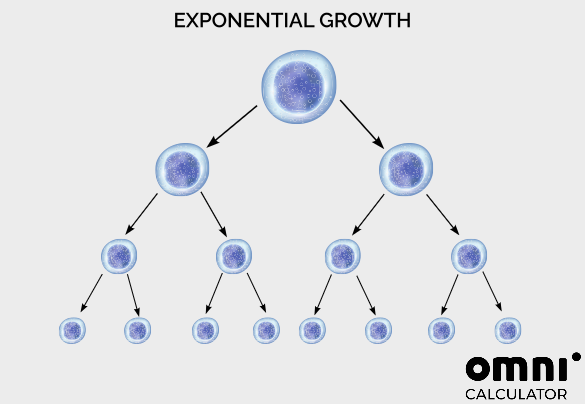# Cell Doubling Time Calculator

Created by Julia Żuławińska
Reviewed by Steven Wooding
Last updated: Jun 25, 2022

With our cell doubling time calculator, you can estimate the doubling time of cell culture using concentration, confluency, or any other suitable parameter. Keep reading on to find out how to calculate the doubling time of cells and learn the cell culture doubling time formula.

The cell doubling time is the time it takes a cell culture to double. Each cell type has its individual doubling time that depends on cell culture conditions. Air and nutrients accessibility, temperature, and pressure are just a few factors that affect cell growth rate.

For example, the bacterium E. coli in a laboratory can divide every 20 minutes (read more about this in our generation time calculator), whereas, in our intestines, where it naturally occurs, it needs several hours. Under certain conditions, one cell type proliferates at a (more or less) constant speed. That proliferation causes an exponential increase in cell number.

Cell doubling has many practical applications, including purifying water in a wastewater plant.## How to calculate doubling time of cells?

To calculate the doubling time of cells, use the following formula:

$\footnotesize \text{Doubling time} = \frac{ \text{Duration}*ln(2)}{ln(\frac{\text{Final concentration}}{\text{Initial concentration}})}$

To use this cell culture doubling time formula, you need to:

1. Select a reference parameter. It can be the number of cells, concentration, or confluency. Measure it at the beginning of an experiment.
2. Wait for a certain period. Depending on the cell type and culture conditions, it can be a few minutes, hours, or days.
3. Check chosen parameter after a suitable period.
4. Calculate the doubling time.

💡 Concentration is the number of cells per unit of volume (i.e., cells/ml). You can find it, for example, by using a hemocytometer like the Bürker counting chamber. Confluency is the percentage coverage of a container surface. This parameter can be found only for adherent cells.

## How to calculate like our cell doubling time calculator?

Let's say you have a cell culture of pancreatic cancer cells, and you need to find the doubling time under certain conditions. You used the Bürker chamber and found that you have 10,400 cells per milliliter. You've waited for 72 hours and found that the concentration has changed to 27,600 cells per milliliter.

To find the doubling time of this cell culture, all you need to do is use the doubling time formula:

$\footnotesize \text{Doubling time} = \frac{ \text{Duration}*ln(2)}{ln(\frac{\text{Final concentration}}{\text{Initial concentration}})}$

where:

• Duration equals 72 hours;
• Initial concentration is 10,400 cells/milliliter; and
• Final concentration is 27,600 cells/milliliter.

💡 ln means the natural logarithm – a logarithm to the base of mathematical constant e.

$\footnotesize \text{Doubling time} = \frac{72*ln(2)}{ln(\frac{27600}{10400})} = \frac{72*0.6931}{ln(2.653846)} = \frac{49.9032}{0.976} = 51.1 \text{hours}$

This cell culture doubling time is equal to around 51 hours. If you also want to find the growth rate, use this formula:

\footnotesize \begin{aligned} \text{Growth rate} &= \frac{ln(\frac{\text{Final concentration}}{\text{Initial concentration}}) }{\text{Duration}} \\ &= \frac{0.976}{72} = 0.01356 \frac{\text{cells}}{\text{hour}} \end{aligned}

The growth rate equals 0.01356 cells per hour.

Interested in cell biology? The protein molecular weight calculator is here to help you with your studies! Also make sure to check our log reduction tool to calculate log and percentage reduction of microorganisms after applying a treatment.

## FAQ

### How to calculate doubling time of bacteria?

Every bacteria population has four characteristic growth phases: lag, exponential growth, stationary, and death. To find the doubling time (also known as generation time) of bacteria, you need to find the concentration (i.e., by measuring the optical density) at the beginning and end of the exponential growth phase. Then, check how long this exponential growth phase was, and use the cell culture doubling time formula:

Bacteria doubling time = Duration × ln(2) / ln(Final concentration / Initial concentration)

### How long does it take for bacteria to double?

Bacteria can double every 10 minutes, every 5 hours, every 60 hours, or even every thousand years. The doubling time of bacteria depends on their type, living environment, and access to nutrients. For example, the bacterium E. coli in a lab can double once every 20 minutes, whereas, in our intestines, where it naturally occurs, it needs several hours.

### What is exponential growth in biology?

Exponential growth in biology is connected to the growth of an organism's population. If an organism of a certain species always makes approximately the same number of offspring (and it's more than one), and it happens in approximately the same time, its population will grow exponentially (if there are abundant resources, space, and no predators).

A perfect example of exponential growth is cell division. Each cell creates two descendant cells. At first, there is one cell, then two, four, eight, sixteen, and so on.

Julia Żuławińska
Initial reference parameter
Final reference parameter
Time duration
hrs
Growth rate
per h
Doubling time
hrs
min
People also viewed…

### Black Friday

How to get best deals on Black Friday? The struggle is real, let us help you with this Black Friday calculator!

### Plant population

Plant population calculator helps you find the number of plants you can grow on your farm field for you to make early yield and profitability estimates.

### Snowman

The perfect snowman calculator uses math & science rules to help you design the snowman of your dreams!

### Water potential

With this water potential calculator you can calculate the effect of the various components of the water potential on the flow of water.